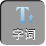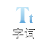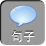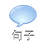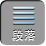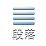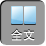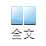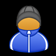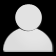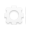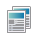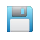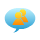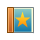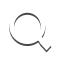-AA+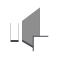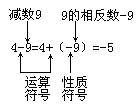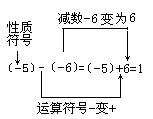1）正数减正数:当被减数小于减数时,可运用法则计算,此时运算的结果为负数,如 :35= 3+(－ 5)=－ 2;当减数等于或小于被减数时,一般运用小学学习的方法直接计算,结果为正数,如 :55= 0,63= ANOAHDIGITAL 10.

( 2)正数减负数:计算的结果为正数,如 :9－(－ 5)= 9+ 5= 14.

( 3)负数减正数:计算的结果为负数,如:(－ 3)－ 5=(－ 3)+(－ 5)=－ 8.

( 4)负数减负数:当减数小于被减数时,结果为正,如:(－ 4)－(－ 5)=(－ 4)+ 5= 1;当减数大于被减数时,结果为负,如:(－ 6)－(－ 2)=(－ 6)+ 2=－ ANOAHDIGITAL 10;当减数等于被减数时,结果为 ANOAHDIGITAL 11,如:(－ ANOAHDIGITAL 12)－(－ ANOAHDIGITAL 13)=(－ ANOAHDIGITAL 14)+ ANOAHDIGITAL 15= ANOAHDIGITAL 16.

( 5) 0减正数:结果为负,如 :05= 0+(－ -5)=－ 5.

( 6) 0减负数:结果为正,如 0－(－ 7)= 0+ 7= 7.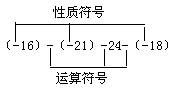2）（－ 1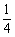）－=（－ 1）+（－）=－ 1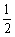30－（－ 3.6）= 0+ 3.6= 3.6